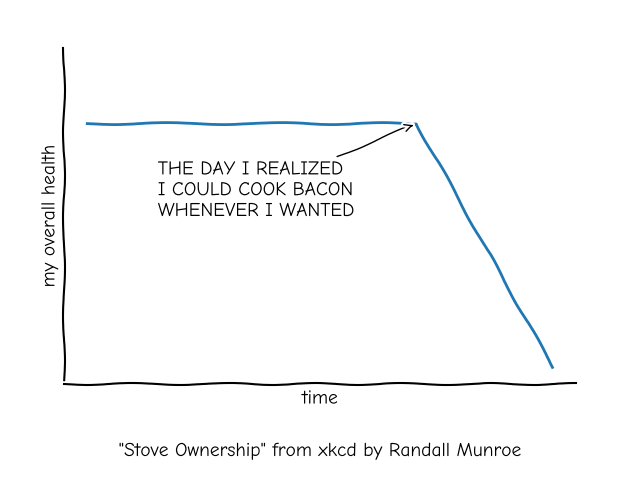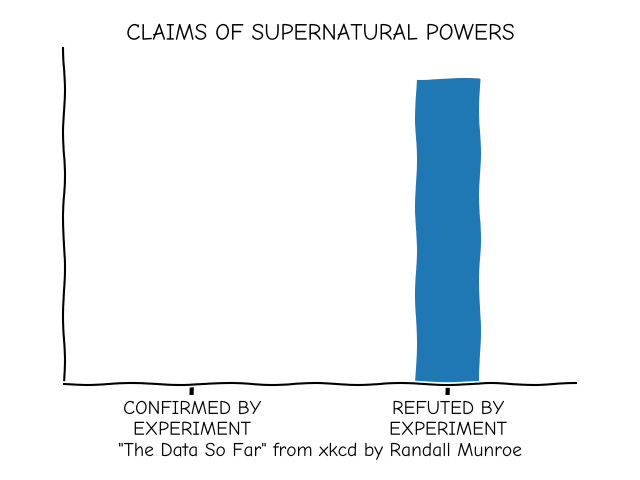# XKCD#

Shows how to create an xkcd-like plot.

import matplotlib.pyplot as plt
import numpy as np

with plt.xkcd():
# Based on "Stove Ownership" from XKCD by Randall Munroe
# https://xkcd.com/418/

fig = plt.figure()
ax = fig.add_axes((0.1, 0.2, 0.8, 0.7))
ax.spines[['top', 'right']].set_visible(False)
ax.set_xticks([])
ax.set_yticks([])
ax.set_ylim([-30, 10])

data = np.ones(100)
data[70:] -= np.arange(30)

ax.annotate(
'THE DAY I REALIZED\nI COULD COOK BACON\nWHENEVER I WANTED',
xy=(70, 1), arrowprops=dict(arrowstyle='->'), xytext=(15, -10))

ax.plot(data)

ax.set_xlabel('time')
ax.set_ylabel('my overall health')
fig.text(
0.5, 0.05,
'"Stove Ownership" from xkcd by Randall Munroe',
ha='center')with plt.xkcd():
# Based on "The Data So Far" from XKCD by Randall Munroe
# https://xkcd.com/373/

fig = plt.figure()
ax = fig.add_axes((0.1, 0.2, 0.8, 0.7))
ax.bar([0, 1], [0, 100], 0.25)
ax.spines[['top', 'right']].set_visible(False)
ax.xaxis.set_ticks_position('bottom')
ax.set_xticks([0, 1])
ax.set_xticklabels(['CONFIRMED BY\nEXPERIMENT', 'REFUTED BY\nEXPERIMENT'])
ax.set_xlim([-0.5, 1.5])
ax.set_yticks([])
ax.set_ylim([0, 110])

ax.set_title("CLAIMS OF SUPERNATURAL POWERS")

fig.text(
0.5, 0.05,
'"The Data So Far" from xkcd by Randall Munroe',
ha='center')

plt.show()Gallery generated by Sphinx-Gallery